Courses

# Relations among AM, GM and HM - Measures of Central Tendency, Business Mathematics & Statistics B Com Notes | EduRev

Created by: Arshit Thakur

## B Com : Relations among AM, GM and HM - Measures of Central Tendency, Business Mathematics & Statistics B Com Notes | EduRev

The document Relations among AM, GM and HM - Measures of Central Tendency, Business Mathematics & Statistics B Com Notes | EduRev is a part of the B Com Course Business Mathematics and Statistics.
All you need of B Com at this link: B Com

Relations among A.M., G.M. and H.M. :

1. The Arithmetic Mean is never less than the Geometric Mean, again Geometric Mean is never less than the Harmonic Mean. i.e. A.M. ≥ G. M. ≥ H. M.

Uses of H.M. : Harmonic mean is useful in finding averages involving rate, time, price and ratio.

Example 23 : For the numbers 2, 4, 6, 8, 10, find GM & HM and show that AM > GM > HM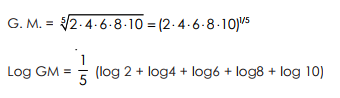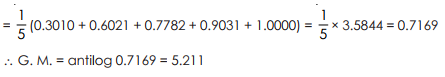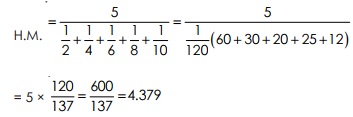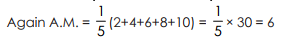We get A.M. =6, G. M. = 5.211, H.M. = 4.379 i.e. A.M. ≥ G. M. ≥ H. M

Note : In only one case the above relation is not true. When all the variates are equal, we will find that AM = GM = HM

Example 24 : A.M. and G.M. of two observations are respectively 30 and 18. Find the observations. Also find H.M.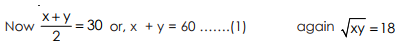Or, xy = 324 or, (60 – y). y = 324, from (1)
Or, y2 – 60 y + 324 = 0 or, (y –54) (y –6) = 0, y = 54, 6
∴y = 54 , x = 6 or, y = 6, x = 54.
∴ Required observations are 6, 54.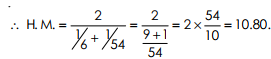Offer running on EduRev: Apply code STAYHOME200 to get INR 200 off on our premium plan EduRev Infinity!

122 videos|142 docs

,

,

,

,

,

,

,

,

,

,

,

,

,

,

,

,

,

,

,

,

,

,

,

,

,

,

,

;